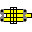#Combinatorics

## Factorial

Factorial is important function widely used in combinatorial formulas. Unfortunately there is no easy way to calculate factorials.

Factorial of number n is denoted n! and is equal 1*2*...*n.

In this chapter log means base 10 log.

### Factorials of small numbers

First of all, for n<7 it is easy just to remember their factorials:
1!=1
2!=2
3!=6
4!=24
5!=120
6!=720

If n<16 I believe the easiest way to calculate factorial is to use chain multiplication: begin with 6! and multiply it consequently by the growing integers. You can skip multiplying by 10 and you can group multiplicands (for example 12!=6!*(63=7*9)*(88=8*11)*120) can be performed with only 2 slide moves.

### Factorial of big numbers (B,C,CI,D,L)

The following assumes that your L scale is located on stator (some rules have it on slide). This is not "simple" operation at all: it requires 3 slide movements and 2 value transfers from scale to scale. But it is the simplest way to calculate factorial I know, and factorial is very important function so it is included here.

The calculations are based on a Stirling formula:

n! ~ √(2πn)*(n/e)n
1. Put e=2.718 on scale C over n on scale D.
2. Read fractional part from L under 1C. (This is fractional part of log(n/e)
3. Add integer part that is dc(n)-1 if you moved slide to the right and dc(n)-2 if you moved slide to the left.
4. Place the cursor on that number on scale D.
5. Place n on scale CI under cursor.
6. Read value under 1CI from D.
7. Set fractional part (if distinguishable) of the result on scale L with a cursor, value on scale D have dc=integer part+1.
8. If the fractional part was beyond slide rule accuracy just add 0.5*dc(n) to the result you get in step 6 and it will be the order of magnitude for the factorial, otherwise continue.
9. Place 0.399 on scale CI under cursor. (0.399=1/√(2π) -- you can always look it up with cursor if you do not remember it)
10. Read n! under n on scale B from scale D.

### When to apply last 2 steps?

When the fractional part becomes undistinguishable? It depends on the accuracy of your slide rule and your scale reading skills. For me and my rule all numbers greater than 200 are integer. So, I will take it as an upper bound for expression n*log(n/e) after which the last 2 steps shouldn't be applied.

The equation n*log(n/e)=200 could not be solved in closed form in elementary functions, but fortunately it can be solved approximately with slide rule. (See Equations chapter for generalization of this method towards other types of equations.)

n*log(n/e)=200 <=>
log(n/e)=200/n <=>
log(n)=200/n+0.434 <=> log(n)-200/n=0.434

Now place (right) index of CI over 200 on scale D. With this layout D represents n (ranging 100 .. 200), L represents log(n) (ranging 2.0 .. 2.3) and CI represents 200/n (ranging 2..1).

With cursor lookup values on scales CI and L that when subtracted give 0.434 as a result. It may be easier to match value on L that equal 0.434+value on C.

Trying CI=1.6: CI+0.434=2.34; L=2.1

Trying CI=1.5: CI+0.434=1.93; L=2.124

The result is between above two values. After couple more tries find CI=1.58; CI+0.434=2.13; L=2.106 (that is exact enough) and read value n=127 on scale D.

This means that for n>127 we can only estimate the order of magnitude for the factorial using this method.

[ Index page | Emulator | Notation | Deframe | Feedback ]